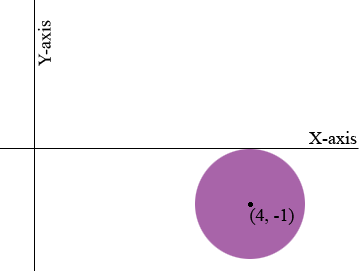SEARCH HOMEMath Central Quandaries & QueriesQuestion from Kiera, a student: Find an equation of the circle that satisfies the stated conditions. Center C(4,-1), tangent to the x-axisHi Kiera,

The equation of a circle with radius r and centre (h, k) is (x - h)2 + (y - k)2 = r2.

A tangent to a circle at a point on the circle is perpendicular to the radius at the same point.What is the perpendicular distance from the centre to the X-axis? What is the radius of the circle?

PennyMath Central is supported by the University of Regina and The Pacific Institute for the Mathematical Sciences.